# Cube of Nature: Evolution of physics and mathematics

Mathematics is the mirror of civilization”

Physics deals with the study and understanding of the physical world. And simultaneous study and measurement of experiment and mathematics lead us to the fundamental understanding of nature. Mathematics is the language of physics, and hence considered as the universal language to understand our existence and nature in this universe. Mathematics represents trust and truth. It may be true that the same mathematical theories hold true in other planets also, so it is a must for a good physicist to have a strong background of mathematics. The human mind, physical world and mathematics relate with each other in a subtle way. It is a universal eternal triangle. And because of this triangle, the quest for knowing more and more about this universe goes on. Stephen Hawking once remarked, “There is no need of invoking God, as long as we have mathematics”.

The human thirst for knowledge started a long time ago. Greek philosophers used to say that the four fundamental forces that constitute the universe were fire, air, water and land. If this theory were to be true, our life would have been simpler. But the quest was never ending. As the mathematical theories developed, more realistic theories were put forward by philosophers. The classical era was started by Kepler’s law. Kepler’s laws (1609) were the starting point in understanding physical world (Astronomia Nova). This was followed by the celebrated Newton’s laws of motion and Newton’s gravitational theory (Principia). Newtonian picture of the world is probably the most famous of all the theories that have been invented or discovered so far. It was the first window to look through nature. Newton used Euclidean geometry to represent his point of view of nature. Newton also started a new frontier in physics by discovering calculus to derive gravitational force equations, which led to the beginning of classical mechanics viz. Lagrangian and Hamiltonian formalisms. On the other side of the history in science, the empirical laws of electromagnetism lead to lines of force due to Faraday (experimentalist) and then to Maxwell’s four equations which are first order differential forms in symmetric space and time co-ordinates (gauge invariance and Lorentz transformation implicit). Also the introduction of Lorentz transformation leads to the geometry of flat space-time and birth of special theory of relativity of Albert Einstein. A more general symmetry (General Covariance for all frames including non-inertial frame) combined with Equivalence Principle (Inertia=Gravity), leads to the development of Einstein’s General Theory of Relativity (GTR) (1916). In GTR the 4-dimentional curved space-time embodies the gravitational force. General Covariance is a local symmetry principle and extra structure known as affine connection (which represents gravitational potential) is needed to satisfy geometry of curved space-time and concept of classical field (Riemannian Geometry).

The classical era was more of a geometrical era, or we can say a macro scale world. But scientific odyssey of atomic scale leads us to the astonishing world that we cannot see with our naked eye. This world is known as the quantum world. Thousands and thousands of probable theories engulfed in physicist’s mind after the discovery of quantum mechanics. In a simple language we can say, if classical era can be represented by x and y axis, then quantum mechanics is z-axis with infinite boundary. We can go deep and deep in the axis, it has infinite probabilities and too much uncertainties. Mathematics plays the most important role in quantum mechanics. Because we directly cannot see or feel anything of the quantum world, so whatever we interpret, we interpret through the language of mathematics. If we can understand the physical interpretation of mathematical formulae, we can try to understand quantum mechanics. Schrodinger once said, ”No one completely understands quantum mechanics”. D.Griffith wrote, “I cannot teach you quantum mechanics, I can only teach the tools to understand quantum mechanics”. Because of this fascinating difficulties and infinite probabilities, quantum mechanics is the most tempting branch of physics. It is only because of the discovery of advanced level mathematics that we can interpret quantum theories. It is based on operators in Hilbert space, functional analysis, linear algebra and infinite dimensional vector spaces, etc. Discrete quantities e.g., energy level, angular momentum etc. are allowed. There are global effects e.g., wave-functions not localized. The theory is probabilistic (hence wave function) and also needs quantization (because of Hermitian operator of observables). Some important equations in quantum mechanics are Schrodinger equation for non-relativistic dynamics, Heisenberg equation in operator form of Hermitian operators, Klein-Gordon equation for relativistic dynamics, Dirac equation for relativistic dynamics, Schwinger-Dyson equation in QFT, The Breit equation for Helium, Bethe-Salpeter equation for bound state systems etc.. These equations have profound applications in diverse areas of theoretical physics. Since science never solves a problem without creating ten more, some inconsistencies in relativistic quantum mechanics led to the discovery of quantum field theory (QFT). We can also interpret QFT as the successful merging of quantum mechanics and special theory of relativity. There is a famous diagram of how evolution of classical mechanics goes to quantum mechanics and quantum field theory. The diagram is shown below: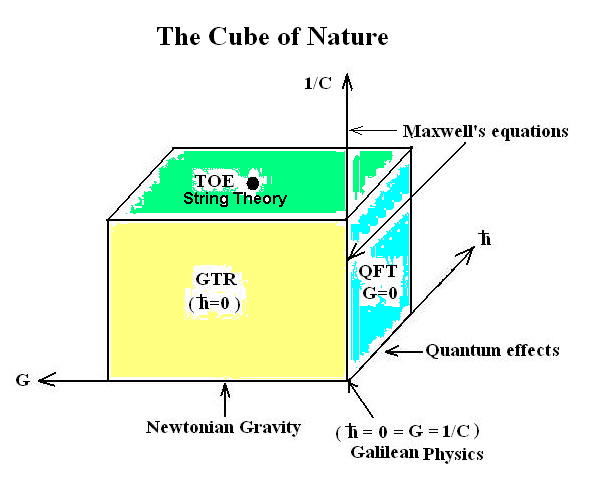We have seen above how the evolutionary journey of mathematics helped us to discover new physical theories to understand the fundamental existence of nature. There is a famous mathematical model for representing all the principal physical models in a cube, known as cube of nature. The frame of reference used to place the cube uses the coordinates in three perpendicular axes viz. Gravitational constant (G), velocity of light (c) and Planck’s constant (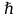). These three quantities behave as fundamental quantities in nature. These constants have importance in physical world. Gravitational constant has universal character, only depends on space dimension being three. Velocity of light plays a decisive role in Maxwell’s equations and special theory of relativity. And Planck’s constant governs the interaction of light and matter, wave particle duality and an important factor in uncertainty principle and commutation relations in quantum mechanics. So, basically if we take G, c and h as fundamental axes, we can express all the physical models.

In the cube, (G=0, h=0, c=0) origin represents the the Newtonian physics. G line represents the Newtonian gravitational theory, h line presents the non-relativistic quantum mechanics, and c line represents Maxwell’s electrodynamics and special theory of relativity. (G, c) surface represents the GTR while (h,c) surface represents the QFT. Probably (G, h,c) surface will represent the final theory of physical world. Using these fundamental quantities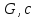and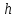, we can understand the very basic units of length, time and mass i.e. Planck’s length, Planck’s time and Planck’s mass, which are considered as the smallest quantities of any measurement that has any meaning. The formulae are shown below: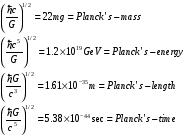In the cube of nature, when a physical quantity involves three quantities as coordinate i.e. (G,c,h), that is the final theory, known as theory of everything or theory of nature. This new theory will encompass both GTR and QFT under the same framework. String theory as a candidate for the theory of everything, has been proposed. In this theory fundamental entities are considered as one-dimensional objects (strings close or open) as to point particle concept in QFT.

This article is just a glimpse to show how mathematics and physics are opposite sides of the same coin. From the very beginning this correlation of mathematics and physics led us to what we have in present. From the very basic theory of fire, land, air, water to the complex theories of string theory and theory of everything. This article shows how important mathematics is for a physicist to understand the concepts in physics. Earlier physicist tried to develop new mathematical theories to put forward their physical theories, but now-a-days physicists are mathematicians. At higher level, physicists and mathematicians share the same plate of bread and butter. This overlapping and collaboration of mathematics and physics is a dire necessity to understand and get a well-established theory of nature.

Learning new tricks in mathematics make me younger”

[This article would have been impossible without my teacher Prof. N. Nimai Singh’s resources and presentation.]